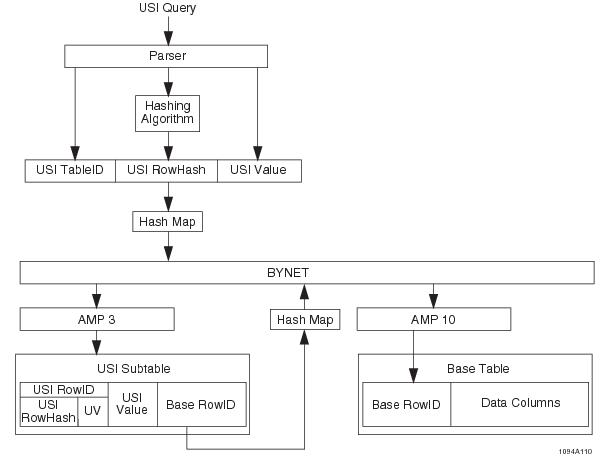# 15.00 - USI Access - Teradata Database

prodname
vrm_release
15.00
category
User Guide
featnum
B035-1094-015K

### USI Access

USI access is usually a two-AMP operation because Teradata Database typically distributes a USI row to a different AMP than the base table row the index points to. If the system distributes the USI subtable row to the same AMP as the base table row it points to, then only one AMP is accessed (but it is still a two-step operation).

The following stages are involved in a USI base table row access.

• The requested USI value is accessed by hashing to its subtable.
• The pointer to the base table row is read and used to access the stored row directly.
• The flow diagram illustrates the following query. Note that table_name is an NPPI table.

`     SELECT *`
`     FROM table_name`
`     WHERE USI_column = value;`The process for locating a row using a USI is as follows.

1 After checking the syntax and lexicon of the query, the Parser looks up the Table ID for the USI subtable that contains the specified USI value.

2 The hashing algorithm hashes the USI value.

3 The Generator creates an AMP step message containing the USI Table ID, USI row hash value, and USI data value.

4 The Dispatcher uses the USI row hash to send the message across the BYNET to AMP 3, which contains the appropriate USI subtable row.

5 The file system on AMP 3 locates the appropriate USI subtable using the USI Table ID.

6 The file system on AMP 3 uses the USI rowID to locate the appropriate index row in the subtable.

This operation might require a search through a number of rows with the same row hash value before the row with the desired value is located.

7 AMP 3 reads the base table rowID from the USI row and distributes a a message containing the base table ID and the rowID for the requested row across the BYNET to AMP 10, which contains the requested base table row.

The distribution is based on the hash bucket value in the rowID of the base table row.

8 The file system uses the rowID to locate the base table row.

### Example

The following example performs a single‑row lookup. The column named cust_num is a USI for the customer table, which is an NPPI table.

`     SELECT name, phone`
`     FROM customer`
`     WHERE cust = 3;`

The example customer table and USI subtable are as follows.

The secondary index value (cust_num) is hashed to generate rowID R9 (note that +2 represents the uniqueness number). The AMP retrieves row R9+2 from the secondary index subtable. The subtable row contains the rowID of the base table row, which can then be accessed.